In C#, it is possible to make operators work with user-defined data types like classes. That means C# has the ability to provide the operators with a special meaning for a data type, this ability is known as operator overloading. For example, we can overload the + operator in a class like String so that we can concatenate two strings by just using +.

Using operator overloading in C# we can specify more than one meaning for an operator in one scope. The purpose of operator overloading is to provide a special meaning of an operator for a user-defined data type.

To overload an operator in C#, we use a special operator function. We define the function inside the class or structure whose objects/variables we want the overloaded operator to work with. The Syntax for Operator Overloading in C# is shown below.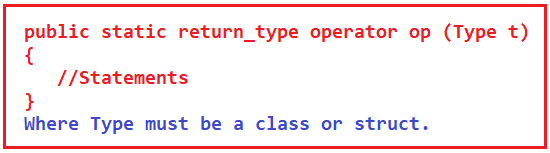Here,

1. The return type is the return type of the function.
2. the operator is a keyword.
3. Op is the symbol of the operator that we want to overload. Like: +, <, -, ++, etc.
4. The type must be a class or struct. It can also have more parameters.
5. It should be a static function.

We have operators for performing addition (+), multiplication (*), subtraction (-), increment and decrement operator (++, –), and so on. That means to perform various kinds of things there are operators available in C#. And these operators are meant for some specific data types. The following table describes the overloading ability of the various operators available in C#: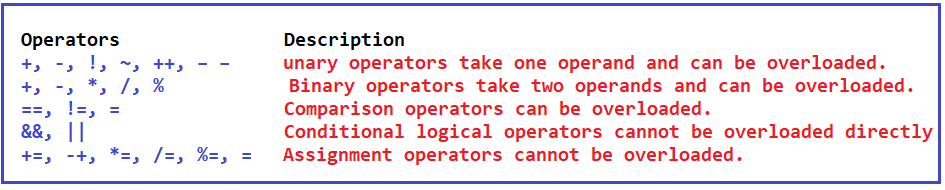The above image shows some built-in operators and these operators are operating on built-in data types or primitive data types available in C#. Like additions can be performed on integer, float, and so on. If we are defining our own data type like if we are writing a class Matrix.

class Matrix {

}

Then can we use the + operator for adding two matrices and store the result in another object of type matrix (C = A + B)? So, can we overload + operator for the matrix class? Yes, + operator can be overloaded for the matrix class.

So, for our own data type which is a user-defined data type, we can overload + operator. There are various operators that you can overload in C#. So, let us learn how to overload these operators.

Let us take an example of a complex number to understand Operator Overloading in C#. In mathematics, we have a complex number that is written in the form of a + ib as shown in the below image.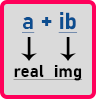Here a is the real part and ib is the imaginary part. What is imaginary? Here, i is the square root of -1.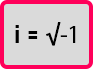The square root of minus one is undefined. So, we call it imaginary (i). Anything multiplied by an imaginary number becomes an imaginary number. Here, a is an integer or float number and ib is imaginary. If we have two complex numbers then we can add them by adding their real part and imaginary part separately. For example, if we have 3 + 7i and 5 + 2i then after addition we will get 8 + 9i. We got this as the addition of two complex numbers.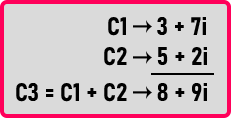Yes, we can perform addition on two complex numbers in mathematics. The same thing we want to achieve programmatically then we want to have + operator overloaded. So let us write a class for a complex number as shown below and see how we can overload the + operator.

```public class Complex
{
private int real;
private int img;
public Complex(int r = 0, int i = 0)
{
real = r;
img = i;
}
};
```

Here, we have created a class called Complex. Inside the Complex class, we have created two integer-type private data members that are real and img. Then we have created a parameterized constructor as public. We can pass the two integer values as parameters to the constructor and the constructor will assign those integer values to real and img private data members of the class.

We have also provided some default values to the constructor arguments so that if the user doesn’t pass any values, then the constructor will automatically assign 0 to real and img data members. This constructor will work as a parameterized constructor as well as a non-parameterized constructor.

Now let us overload + operator. For learning operator overloading we have to learn two things. First, how to write a function, and second, what should be the signature of a function. Signature of a function we will show you afterward, first, let us see how to write a function.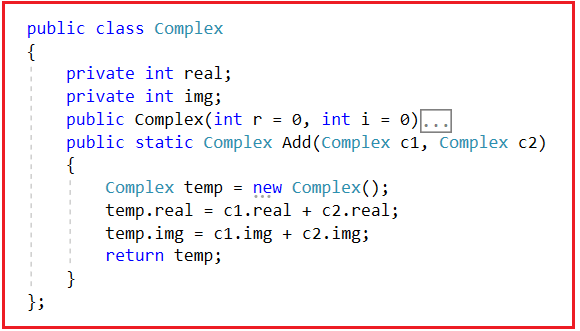Here inside the Complex class, we have written Add function and the return type of this function is Complex. This function will add the real and img values of two Complex objects. Now let us write the main function as follows: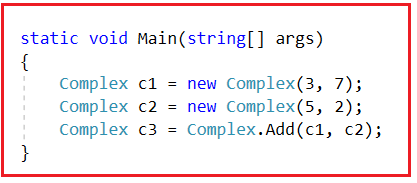Here inside the main function, we have created two objects C1 and C2 of class Complex. C1 will have the values of 3 and 7 and C2 will have the values of 5 and 2. Then we have called the Add function by passing C1 and C2 objects and as this method returns an object of type Complex, so we are storing that object in the C3 reference variable.

##### How Add function is working?

Now let us understand how the add function is working.

In the above statement, we called the static Add function using the class name by passing C1 and C2 as parameters. Once we call the Add method, then the Add method starts executing the code as follows. Inside the Add function, we are creating a temporary complex object by executing the below statement.
Complex temp = new Complex();

Then, we execute the below statement.
temp.real = c1.real + c2.real;

This statement will store the addition of values of C1’s real and C2’s real in temp’s real. Then, the following statement will be executed.
temp.img = c1.img + c2.img;

The above statement will store the addition of values of C1’s img and C2’s img in temp’s img. Finally, we return the temp object from the Add method by executing the below return statement.
return temp;

Then we have returned the temp object from the function. We can understand the above statement with the help of the below diagram.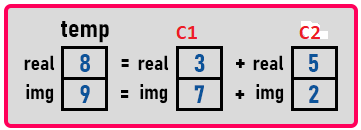This diagram represents that we have stored the result of the addition of C1 and C2 into the temp variable which is of a Complex type. The temp will be returned by the Add function. So inside the main function, we just store the temp data in the C3 object. For a better understanding, please have a look at the below image.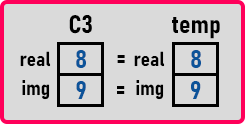So, this is how the addition is done of two complex numbers in C#. So, this logic is important. How to write a function is important.

##### Complete Example to add two Complex Numbers in C#:
```using System;
{
class Program
{
static void Main(string[] args)
{
Complex c1 = new Complex(3, 7);
c1.Display();
Complex c2 = new Complex(5, 2);
c2.Display();
c3.Display();
}
}

public class Complex
{
private int real;
private int img;
public Complex(int r = 0, int i = 0)
{
real = r;
img = i;
}
public static Complex Add(Complex c1, Complex c2)
{
Complex temp = new Complex();
temp.real = c1.real + c2.real;
temp.img = c1.img + c2.img;
return temp;
}
public void Display()
{
Console.WriteLine(\$"{real} + i{img}");
}
};
}
```
###### Output: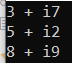Understanding logic is the most important thing. So, we have finished it. Now let us see how to make it as operator overloading. Now we want to convert Add function into an operator. So, instead of writing Complex c3 = Complex.Add(c1, c2);, we want to write Complex c3 = C2 + C1;

So, for writing like this, we have to modify the function signature as follows:

public static Complex operator +(Complex c1, Complex c2){}

Here, we just replace the Add word with operator +. For a better understanding, please have a look at the below image.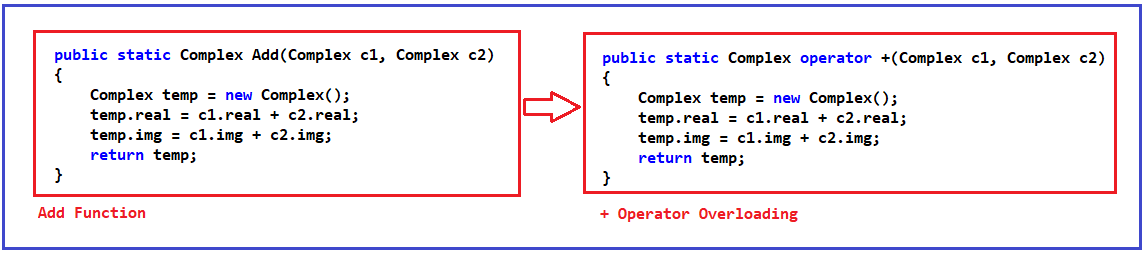Everything inside the function will remain the same. With the above changes in place, now the + operator is overloaded for class Complex. This is Operator Overloading in C#. So instead of writing dot (.), you can just write ‘+’ to get the addition of two Complex objects. Now let us look at the complete program for operator overloading in C#.

```using System;
{
class Program
{
static void Main(string[] args)
{
Complex c1 = new Complex(3, 7);
c1.Display();
Complex c2 = new Complex(5, 2);
c2.Display();
Complex c3 = c1 + c2;
c3.Display();
}
}

public class Complex
{
private int real;
private int img;
public Complex(int r = 0, int i = 0)
{
real = r;
img = i;
}
public static Complex operator +(Complex c1, Complex c2)
{
Complex temp = new Complex();
temp.real = c1.real + c2.real;
temp.img = c1.img + c2.img;
return temp;
}
public void Display()
{
Console.WriteLine(\$"{real} + i{img}");
}
};
}
```
###### Output:Note: In C#, the Operator functions are the same as normal functions. The only difference is, that the name of an operator function is always the operator keyword followed by the symbol of the operator, and operator functions are called when the corresponding operator is used.

1.2.3.